### Triangles Class 9th Mathematics Part Ii MHB Solution

##### Triangles Class 9th Mathematics Part Ii MHB Solution

###### Practice Set 3.1
Question 1.

In figure 3.8, ∠ACD is an exterior angle of ΔABC. ∠B = 40°, ∠A = 70°. Find the measure of ∠ACD.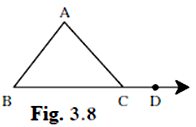Given, ∠A = 70° and ∠B = 40°

In a triangle ABC,

The measure of an exterior angle of a triangle is equal to the sum of its remote interior angles

∠ACD is an exterior angle of triangle ABC

So, from theorem of remote interior angles,

∠ACD = ∠BAC + ∠ABC

⇒ ∠ACD = ∠A + ∠B

⇒ ∠ACD = 70° + 40° = 110°

Question 2.

In ΔPQR, ∠P = 70° ∠Q = 65° then find ∠R.

Given, ∠P = 70° ∠Q = 65°

In a triangle we know sum of interior angles is 180°

∴ in ΔPQR

∠P + ∠Q + ∠R = 180°

70° + 65° + ∠R = 180°

∠R = 180° - 135° = 45°

Question 3.

The measures of angles of a triangle are x°, (x – 20)°, (x – 40)°. Find the measure of each angle.

Question 4.

The measure of one of the angles of a triangle is twice the measure of its smallest angle and the measure of the other is thrice the measure of the smallest angle. Find the measures of the three angles.

Let the measure of the smallest angle be x

Measure of second angle = 2x

Measure of third angle = 3x

In a triangle we know sum of interior angles is 180°

∴ x + 2x + 3x = 180°

⇒ 6x = 180°

⇒ x = 180°/6

⇒ x = 30°

Measure of smallest angle = x = 30°

Measure of second angle = 2x = 2 × 30° = 60°

Measure of third angle = 3x = 3 × 30° = 90°

Question 5.

In figure 3.9, measures of some angles are given. Using the measures find the values of xyz.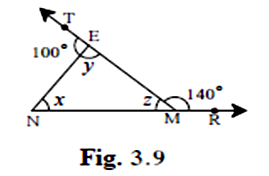Given ∠TEN = 100°, ∠EMR = 140°

∠NEM = y, ∠ENM = x, ∠NME = z

In a triangle ENM

The measure of an exterior angle of a triangle is equal to the sum of its remote interior angles

∠TEN and ∠EMR is an exterior angle of triangle ENM

So from theorem of remote interior angles,

∠TEN = ∠ NME + ∠ENM

⇒ 100° = z + x ……. (1)

∠EMR = ∠NEM + ∠ENM

⇒ 140° = x + y

⇒ x = 140° - y …(2)

In a triangle we know sum of interior angles is 180°

∴ x + y + z = 180 ……….(3)

Putting (1) in (3)

⇒ y + 100° = 180°

⇒ y = 180° - 100° = 80°

Putting y in (2)

∴ x = 140° - 80°

⇒ x = 60°

Putting x in (1)

∴ 60° + z = 100°

⇒ z = 100° - 60°

⇒ z = 40°

Measure of all the angles are

x = 60°, y = 80°, z = 40°

Question 6.

In figure 3.10, line AB || line DE. Find the measures of ∠DRE and ∠ARE using given measures of some angles.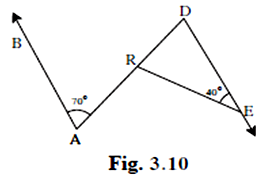Given ∠DAB = 70° and ∠DER = 40°

In the given figure ∠DAB = ∠ADE [Alternate Interior angles are equal]

∴ ∠ADE = ∠RDE = 70°

In ΔDER,

∠DER + ∠DRE + ∠RDE = 180°

⇒ 40° + ∠DRE + 70° = 180°

⇒ ∠DRE = 180° - 110°

⇒ ∠DRE = 70°

∵ ∠ARE is an exterior angle of triangle DER

∠ARE = ∠RDE + ∠DER = 70° + 40°

⇒ ∠ARE = 110°

Question 7.

In ΔABC, bisectors of ∠A and ∠B intersect at point O. If ∠C= 700 Find measure of ∠AOB.

The figure is attached below:

BN and AM are the angle bisectors of angle B and A respectively.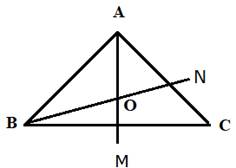Given ∠C = 70°

In a triangle we know sum of interior angles is 180°

In ΔABC

∠A + ∠B + ∠C = 180°

∠A + ∠B = 180° - 70°

∠A + ∠B = 110°

Now in ΔAOB

AO is the bisector of ∠A

BO is the bisector of ∠B

∴ ∠OAB = ∠A/2 and ∠OBA = ∠B/2

∠OAB + ∠OBA + ∠AOB = 180°

∠A/2 + ∠B/2 + ∠AOB = 180°

⇒ ∠AOB = 180° - (∠A + ∠B)/2

⇒ ∠AOB = 180° - 110°/2 = 180° - 55°

⇒ ∠AOB = 125°

Question 8.

In Figure 3.11, line AB || line CD and line PQ is the transversal. Ray PT and ray QT are bisectors of ∠BPQ and ∠PQD respectively.

Prove that ∠PTQ = 90°.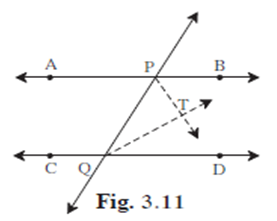Given: AB || CD, line PQ is the tranversal

Ray PT and Ray QT are bisectors of ∠BPQ and ∠PQD

To prove: ∠PTQ = 90°

Proof: Since, Ray PT and Ray QT are bisectors of ∠BPQ and ∠PQD

∠TPQ = ∠BPQ/2 ……..(1)

∠PQT = ∠PQD/2 ………(2)

Since, two parallel lines are intersected by a transversal, the interior angles on either side of the transversal are supplementary.

So, ∠BPQ + ∠PQD = 180°

Dividing both sides by 2, we get

⇒ (∠BPQ + ∠PQD)/2 = 180°/2

⇒ ∠BPQ/2 + ∠PQD/2 = 90°

In ΔPQT,

∠TPQ + ∠PQT + ∠PTQ = 180°

Substituting ∠TPQ and ∠PQT from (1) and (2) respectively

⇒ ∠BPQ/2 + ∠PQD/2 + ∠PQT = 180°

⇒ 90° + ∠PQT = 180°

⇒ ∠PQT = 180° - 90°

⇒ ∠PQT = 90°

Hence, proved.

Question 9.

Using the information in figure 3.12, find the measures of ∠a, ∠b and ∠c.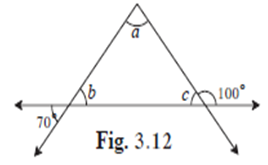In the given triangle

a + b + c = 180° …………(1)

c + 100° = 180° ……….(2) [angles in linear pair]

⇒ c = 180° - 100°

⇒ c = 80°

b = 70° ……………..(3) [opposite angles are equal]

Putting value of b and c in (1)

⇒ a + 70° + 80° = 180°

⇒ a = 180° - 150°

⇒ a = 30°

Question 10.

In figure 3.13, line DE || line GF ray EG and ray FG are bisectors of ∠DEF and ∠DFM respectively.

Prove that,

i. ∠DEG = 1/2∠EDF

ii. EF =FG.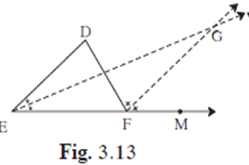Given: line DE || line GF

Ray EG and ray FG are bisectors of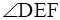and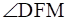respectively

To Prove: i.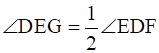ii.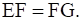Proof: Ray EG and ray FG are bisectors ofand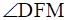respectively.

So, ∠DEG = ∠GEF = 1/2 ∠DEF ……………..(1)

∠DFG = ∠GFM = 1/2 ∠DFM ………..(2)

Also, ∠EDF = ∠DFG …..(3) [Alternate interior angles]

In ΔDEF

∠DFM = ∠DEF + ∠EDF

From (2) and (3)

2∠EDF = ∠DEF + ∠EDF

⇒ ∠EDF = ∠DEF

From (1)

⇒ ∠EDF = 2∠DEG

⇒ ∠DEG = 1/2 ∠EDF

Hence, (i) is proved.

Line DE || line GF

From alternate interior angles

∠DEG = ∠EGF …….(4)

From (1)

∠GEF = ∠EGF

Since, in the ΔEGF sides opposite to equal angles are equal.

∴ EF = FG

Hence, (ii) is proved.

###### Practice Set 3.2
Question 1.

In each of the examples given below, a pair of triangles is shown. Equal parts of triangles in each pair are marked with the same signs. Observe the figures and state the test by which the triangles in each pair are congruent.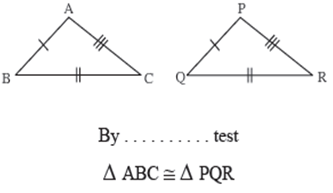: By SSS congruency test

ΔABC ≅ ΔPQR

Explanation:

Given, AB = PQ

BC = QR

CA = RP

∴ By SSS congruency test

ΔABC ≅ ΔPQR

SSS : Side Side Side

Question 2.

In each of the examples given below, a pair of triangles is shown. Equal parts of triangles in each pair are marked with the same signs. Observe the figures and state the test by which the triangles in each pair are congruent.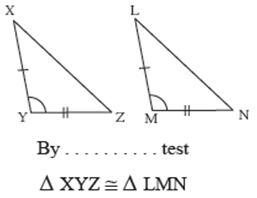By SAS congruency test

ΔXYZ ≅ ΔLMN

Explanation:

Given: XY = LM

∠XYZ = ∠LMN

YZ = MN

Therefore, By SAS congruency test

ΔXYZ ≅ ΔLMN

SAS: Side Angle Side

Question 3.

In each of the examples given below, a pair of triangles is shown. Equal parts of triangles in each pair are marked with the same signs. Observe the figures and state the test by which the triangles in each pair are congruent.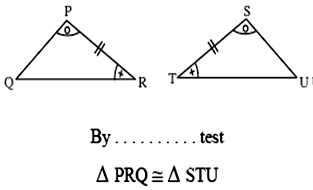By ASA congruency test

ΔPRQ ≅ ΔSTU

Explanation:

Given: ∠QPR = ∠UST

PR = ST

∠PRQ = ∠STU

Therefore, By ASA congruency test

ΔPRQ ≅ ΔSTU

ASA: Angle Side Angle

Question 4.

In each of the examples given below, a pair of triangles is shown. Equal parts of triangles in each pair are marked with the same signs. Observe the figures and state the test by which the triangles in each pair are congruent.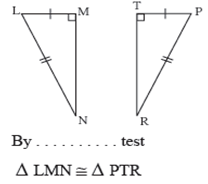By RHS congruency test

ΔLMN ≅ ΔPTR

Explanation:

Given: LM = PT

∠LMN = ∠PTR

LN = PR

Therefore, By RHS congruency test

ΔLMN ≅ ΔPTR

RHS: Right Hypotenuse Side

Question 5.

Observe the information shown in pairs of triangles given below. State the test by which the two triangles are congruent. Write the remaining congruent parts of the triangles.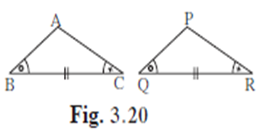From the information shown in the figure, in ΔABC and ΔPQR

∠ABC ≅ ∠PQR

seg BC ≅ seg QR

∠ABC = ∠PRQ

∴ ΔABC ≅ ΔPQR ……………………____ test

∴ ∠BAC = _____……………………corresponding angles of congruent triangles.

seg AB ≅ ____………………….. corresponding sides of congruent triangles.

____ = seg PR …………………corresponding side of congruent triangles.

Given: ∠ABC = ∠PQR

BC = QR

∠ABC = ∠PRQ

∴ ΔABC ≅ ΔPQR …………………………ASA test

ASA: angle side angle

∴ ∠BAC = ∠QPR …………………….corresponding angles of congruent triangles.

seg AB = seg PQ ………………….. corresponding sides of congruent triangles.

seg AC = seg PR ………………….. corresponding angles of congruent triangles.

Question 6.

Observe the information shown in pairs of triangles given below. State the test by which the two triangles are congruent. Write the remaining congruent parts of the triangles.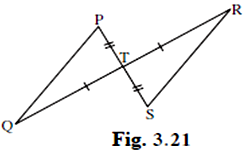From the information shown in the figure.,

In ΔPTQ and ΔSTR

∠PTQ = ∠STR ……………………….. vertically opposite angles

seg TQ ≅ seg TR

∴ ΔPTQ ≅ ΔSTR ………………._____ test

∠TPQ ≅ ____ …………………… corresponding angles of congruent triangles.

_____ ≅ ∠TRS ………………….. corresponding angles of congruent triangles.

seg PQ ≅ _____ …………….. corresponding sides of congruent triangles.

In ΔPTQ and ΔSTR

Given: ∠PTQ = ∠STR ……………………….. vertically opposite angles

seg TQ = seg TR

seg TP = seg TS

∴ ΔPTQ = ΔSTR ……………….SAS test

SAS: side angle side

∠TPQ = ∠TSR …………………… corresponding angles of congruent triangles.

∠TQP = ∠TRS ………………….. corresponding angles of congruent triangles.

seg PQ = seg SR …………….. corresponding sides of congruent triangles.

Question 7.

From the information shown in the figure, state the test assuring the congruence of ΔABC and ΔPQR Write the remaining congruent parts of the triangles.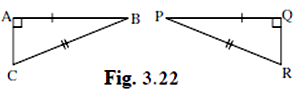In ΔABC and ΔPQR

AB = QP

BC = PR

∠CAB = ∠RQP

∴ By RHS congruency test

ΔABC ≅ ΔQPR

∴ AC = QR ……………….. corresponding sides of congruent triangles.

∠ABC = ∠ QPR ………………… corresponding angles of congruent triangles.

∠BCA = ∠PRQ ………………… corresponding angles of congruent triangles.

Question 8.

As shown in the following figure, in ΔLMN and ΔPMN, LM = PN, LN = PM. Write the test which assures the congruence of the two triangles. Write their remaining congruent parts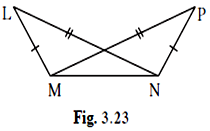Given, In ΔLMN and ΔPNM

LM = PN

LN = PN

MN = MN

∴ By SSS congruency test

ΔLMN ≅ ΔPNM

∠LMN = ∠ PNM ………………… corresponding angles of congruent triangles.

∠LNM = ∠PMN ………………… corresponding angles of congruent triangles.

∠NLM = ∠MPN ……………….. corresponding angles of congruent triangles.

Question 9.

In figure 3.24, seg AB ≅ seg CB and seg AD ≅ seg CD.

Prove that ΔABD ≅ ΔCBD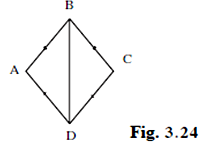Given, In ΔABD and ΔCBD

AB = CB

BD = BD ……….[Common]

∴ By SSS congruency test

ΔABD ≅ ΔCBD

Question 10.

In figure 3.25, ∠P ≅ ∠R seg PQ ≅ seg RQ

Prove that, ΔPQT ≅ ΔPQS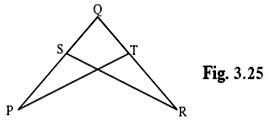In ΔPQT and ΔRQS

∠P = ∠R …………[Given]

∠QPT = ∠QRS

PQ = RQ ………….[Given]

∠PQT = ∠RQS ………….[common]

∴ By ASA congruency

ΔPQT ≅ ΔRQS

###### Practice Set 3.3
Question 1.

Find the values of x and y using the information shown in figure 3.37. Find the measure of ∠ABC and ∠ACB.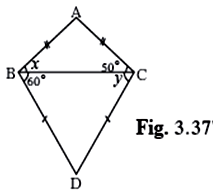In ΔABC

Given, AB = AC

Sides of a triangle are Equal then the angles opposite to them are equal.

∠ABC = ∠ACB

∴ x = 50°

So, ∠ABD = 50° + 60° = 110°

In ΔDBC

Given, DB = DC

Sides of a triangle are Equal then the angles opposite to them are equal.

∠DBC = ∠DCB

∴ y = 60°

∠ACD = ∠ACB + ∠BCD

= 50° + 60°

∴ ∠ACD = 110°

Question 2.

The length of hypotenuse of a right-angled triangle is 15. Find the length of median of its hypotenuse.

Length of hypotenuse of right-angled triangle = 15

We know, the length of the median of the hypotenuse is half the length

of the hypotenuse.

i.e.

Length of median of its hypotenuse = 1/2 × length of hypotenuse

Length of median of its hypotenuse = 1/2 × 15

= 7.5

∴ Length of median of its hypotenuse is 7.5

Question 3.

In ΔPQR, ∠Q =900, PQ = 12, QR = 5 and QS is a median. Find t(QS).

ΔPQR is a right-angled triangle

So, PQ and QR are the sides and PR is the hypotenuse of ΔPQR.

∴ By Pythagoras theorem

PQ2 + QR2 = PR2

⇒ PR2 = 122 + 52 = 144 + 25 = 169

⇒ PR = 13

Length of hypotenuse of right-angled triangle = 13

We know, the length of the median of the hypotenuse is half the length

of the hypotenuse.

i.e.

Length of median of its hypotenuse = 1/2 × length of hypotenuse

Length of median of its hypotenuse = 1/2 × 13

= 6.5

∴ Length of median of its hypotenuse is 6.5

Question 4.

In figure 3.38, point G is the point of concurrence of the medians of ΔPQR. If GT = 2.5, find the lengths of PG and PT.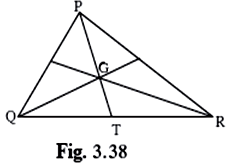Given, in ΔPQR

GT = 2.5

The point of concurrence of medians of a triangle divides each median in

the ratio 2 : 1.

Since, PT is the median.

∴ PG: GT = 2: 1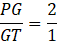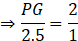⇒ PG = 2 × 2.5 = 5

Therefore, length of PG = 5

Length of PT = PG + GT

= 5 + 2.5

Length of PT = 7.5

###### Practice Set 3.4
Question 1.

In figure 3.48, point A is on the bisector of ∠XYZ. If AX = 2cm then find AZ.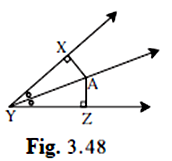Given, Point A is on the bisector of ∠XYZ

AX = 2cm

Every point on the bisector of an angle is equidistant from the sides of the

angle.

Therefore, from figure

AX = AZ

∴ AZ = 2 cm

Question 2.

In figure 3.49, ∠RST = 56°, seg PT ⊥ ray ST, seg PT ⊥ ray ST, seg PR ⊥ ray SR and PR ≅ seg PT Find the measure of ∠RSP. State the reason for your answer.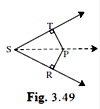Given, ∠RST = 56°

PT perpendicular to ST

PR perpendicular to SR

PR ≅ PT

Since, PR ≅ PT

∴ Any point equidistant from sides of an angle is on the bisector of the

angle.

Therefore, Ray SP is the bisector of ∠TSR.

That is ∠RSP = ∠TSP

Now, ∠RST = ∠RSP + ∠TSP

= 2 ∠RSP

∠RSP = 1/2 ∠RST

∠RSP = 1/2 × 56°

Therefore, ∠RSP = 28°

Question 3.

In ΔPQR, PQ = 10 cm, QR = 12 cm, PR = 8 cm. Find out the greatest and the smallest angle of the triangle.

Given, in ΔPQR, PQ = 10 cm, QR = 12 cm, PR = 8 cm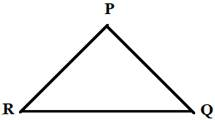We know, If two sides of a triangle are unequal, then the angle opposite to

the greater side is greater than angle opposite to the smaller side.

Here greater side is PQ and the smallest side is PR

∴ Angle opposite to QR = ∠QPR

Angle opposite to PR = ∠PQR

Greatest angle of triangle = ∠QPR

Smallest angle of triangle = ∠PQR

Question 4.

In ΔFAN, ∠F = 80°, ∠A = 40°. Find out the greatest and the smallest side of the triangle. State the reason.

Given In ΔFAN,

∠F = 80°, ∠A = 40°

In a triangle sum of interior angles of the triangle is 180°

∴ ∠F + ∠A + ∠N = 180°

⇒ 80° + 40° + ∠N = 180°

⇒ ∠N = 180° - 120°

⇒ ∠N = 60°

So, ∠F = 80°, ∠N = 60°, ∠A = 40°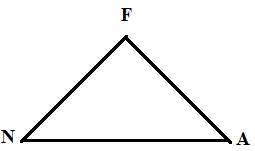If two angles of a triangle are unequal then the side opposite to the greater.

Angle is greater than the side opposite to smaller angle.

Here greatest angle is ∠F and the smallest angle is ∠A

Side opposite to ∠F = NA

Side opposite to ∠A = FN

Greatest side of triangle = NA

Smallest side of triangle = FN

Question 5.

Prove that an equilateral triangle is equiangular

Given: Equilateral triangle PQR

To Prove: ∠P ≅ ∠Q ≅∠R

Proof: PQ ≅ PR ……….[all sides of an equilateral triangle are congruent.]

∠Q ≅ ∠R [the angles opposite to the two congruent sides of a triangle are congruent (Isosceles Triangle Theorem)]

PQ ≅ QR [since all sides of an equilateral triangle are congruent.]

∠R ≅ ∠P, again, by the Isosceles Triangle Theorem

Now, since ∠Q ≅ ∠R and ∠R ≅ ∠P ,

So, ∠Q ≅ ∠P

Therefore, ∠P ≅ ∠Q.

So, equilateral triangles are equiangular.

Question 6.

Prove that, if the bisector of ∠BAC of ΔABC is perpendicular to side BC, then ΔABC is an isosceles triangle.

Given: Bisector of ∠BAC of ΔABC is perpendicular to side BC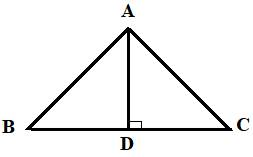To Prove: ΔABC is an isosceles triangle.

Proof:

In ΔABD and ΔACD

Since, AD is the angle Bisector of ΔABC

So, by ASA congruency test

ΔABD ≅ ΔACD

Therefore,

AB = AC ………………. corresponding sides of congruent triangles.

∠ABD = ∠ACD ……………… corresponding angles of congruent triangles.

∴ ∠ABC = ∠ACB

Since, AB = AC and ∠ABC = ∠ACB so, ΔABC is an isosceles triangle.

Question 7.

In figure 3.50, if seg PR ≅ seg PQ, show that seg PS > seg PQ.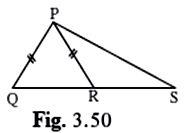Given: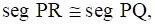To prove: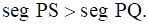Proof:

In ΔPRQ

PQ = PR …………….[given]

∠R = ∠PQ ....(i) [Angles opposite to equal sides are equal]

∠PQR > ∠S …(ii) [exterior angle of a triangle is greater than each of the opposite interior angles]

From (i) and (ii)

∠R > ∠S

PS > PR [side opposite to greater angle is longer]

⇒ PS > PQ [∵ PQ = PR]

Question 8.

In figure 3.51, in ΔABC, seg AD and seg BE are altitudes and AE = BD.

Prove that seg AD ≅ seg BE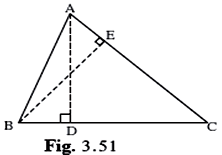Given: AD and BE are altitudes

AE = BD

Proof: AD and BE are altitudes

∠ADB = ∠BEA = 90° [Given]

BD = AE [Given]

∠ADB = ∠BEA = 90° [Given]

AB = BA [Common side of both the triangles]

∴ By RHS congruency

So, AD ≅ BE [corresponding sides of congruent triangles]

###### Practice Set 3.5
Question 1.

If ΔXYZ ∼ ΔLMN write the corresponding angles of the two triangles and also write the ratios of corresponding sides.

Given, ΔXYZ ∼ ΔLMN

Corresponding angles of the two triangles are

∠X = ∠L

∠Y = ∠M

∠Z = ∠N

Ratios of corresponding sides.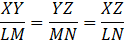Question 2.

In In ΔXYZ, XY = 4 cm, YZ = 6 cm, XZ = 5 cm, If ΔXYZ ∼ ΔPQR and PQ = 8 cm then find the lengths of remaining sides of ΔPQR.

Given,

In ΔXYZ, XY = 4 cm, YZ = 6 cm, XZ = 5 cm

ΔPQR, PQ = 8 cm

ΔXYZ ∼ ΔPQR

So, Ratios of corresponding sides.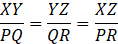⇒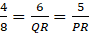⇒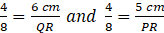⇒ QR = 6 × 2 cm and PR = 5 × 2 cm

⇒ QR = 12 cm and PR = 10 cm

Question 3.

Draw a sketch of a pair of similar triangles. Label them. Show their corresponding angles by the same signs. Show the lengths of corresponding sides by numbers in proportion.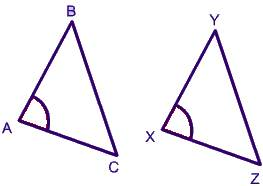ΔABC ∼ ΔXYZ

Corresponding Angles

∠A = ∠X

∠B = ∠Y

∠C = ∠Z

Corresponding Sides in proportion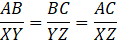###### Problem Set 3
Question 1.

Choose the correct alternative answer for the following questions.

If two sides of a triangle are 5 cm and 1.5 cm, the length of its third side cannot be ………..
A. 3.7 cm

B. 4.1 cm

C. 3.8 cm

D. 3.4 cm

The difference between two sides is less than third side
5 – 1.5 = 3.5

So, the third side cannot be 3.4 cm

Question 2.

Choose the correct alternative answer for the following questions.

In ΔPQR, If ∠R > ∠Q then ………….
A. QR>PR

B. PQ>PR

C. PQ<PR

D. QR<PR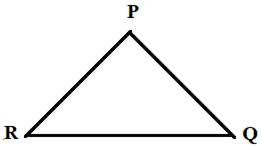∠R > ∠Q

∴ PQ > PR

Question 3.

Choose the correct alternative answer for the following questions.

In ΔTPQ, ∠T = 650, ∠P = 950 which of the following is a true statement?
A. PQ<TP

B. PQ<TQ

C. TQ<TP<PQ

D. PQ<TP<TQ

Sum of interior angles of a triangle = 180°

∠T + ∠P + ∠Q = 180°

⇒ 65° + 95° + ∠Q = 180°

⇒ ∠Q = 180° - 160° = 20°

Since, side opposite to greater angle is greater

∴ TP < PQ < TQ

Question 4.

ΔABC is isosceles in which AB = AC. seg BD and seg CE are medians. Show that BD = CE.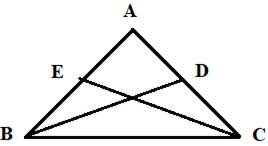Given: ΔABC is an isosceles triangle.

BD and CE are medians.

AB = AC

1/2 AB = 1/2 AC

Since, 1/2 AB = BE = AE and 1/2 AC = AD = CD

So, BE = CD ………….(1)

Also, ∠ABC = ∠ACB

⇒ ∠EBC = ∠DCB ……….(2)

In ΔEBC and ΔDCB

BE = CD [from (1)]

∠EBC = ∠DCB [from (2)]

BC = CB [common side]

∴ By SAS congruency

ΔEBC ≅ ΔDCB

So,

CE = BD …………..corresponding sides of congruent triangles.

∴ BD = CE

Question 5.

In ΔPQR, If PQ>PR and bisectors of ∠Q and ∠R intersect at S. Show that SQ>SR.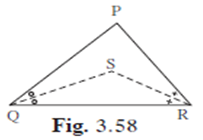Given:

SQ and SR are bisectors of ∠Q and ∠R which meet at S

PQ > PR

To Prove: SQ > SR

Proof:

PQ > PR

∠PRQ > ∠PQR [angle opposite to longer side is larger] …………(1)

SQ and SR are bisectors of ∠Q and ∠R

∴ ∠SQR = 1/2 ∠PQR and ∠SRQ = 1/2 ∠PRQ

Dividing (1) by 1/2 we get

1/2 ∠PRQ > 1/2 ∠PQR

⇒ ∠SRQ > ∠SQR

⇒ SQ > SR [sides opposite to greater angle is longer]

Question 6.

In figure 3.59, point D and E are on side BC of ΔABD, such that BD = CE and AD = AE. Show that ΔABD ≅ ΔACE.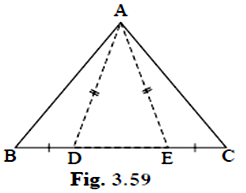Given: BD = CE

To Prove: ΔABD ≅ ΔACE

Proof:

⇒ ∠ADE = ∠AED [angles opposite to equal sides are equal] ……(1)

Subtracting 180° from (1)

⇒ 180° - ∠ADE = 180° - ∠AED

In ΔABD and ΔACE

BD = CE [Given]

∴ By SAS congruency test

ΔABD ≅ ΔACE

Question 7.

In figure 3.60, point S is any point on side QR of ΔPQR.

Prove that: PQ + QR + RP > 2PS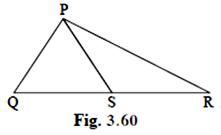Given: S is any point on side QR of ΔPQR.

To Prove: PQ + QR + RP > 2PS

Proof:

We know, sum of two sides of triangle is greater than the third side

∴ In ΔPQS

PQ + QS > PS …………(1)

In Δ PSR

PR + SR > PS ……..(2)

PQ + QS + PR + SR > PS + PS

⇒ PQ + QS + SR + PR > 2PS

⇒ PQ + QR + PR > 2PS [QR = QS + SR]

Hence, proved.

Question 8.

In figure 3.61, bisector of ∠BAC intersects side BC at point D.

Prove that AB > BD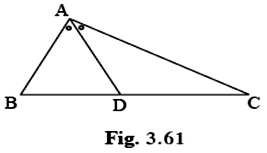Given: AD is bisector of ∠BAC

To Prove: AB > BD

Proof: AD is bisector of ∠BAC

∠ADB > ∠DAC ..(2) [exterior angle of a triangle is greater than each

of the opposite interior angles]

Substituting ∠DAC = ∠BAD in (2)

⇒ AB > BD [side opposite to larger angle is larger]

Question 9.

In figure 3.62, seg PT is the bisector of ∠QPR. A line through R intersects ray QP at point S. Prove that PS = PR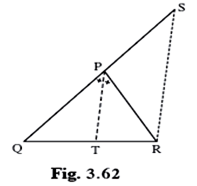Given: PT is angle bisector of ∠QPR

⇒ ∠QPT = ∠RPT

A line through R parallel to PT intersects ray QP at S

RS || PT

To Prove: PS = PR

Proof:

PT is angle bisector of ∠QPR

⇒ ∠QPT = ∠RPT

∠QPR = ∠QPT + ∠RPT

∠QPR = 2∠RPT (1)

RS || PT, PR is the transversal

So, ∠RPT = ∠PRS [Alternate interior angles] (2)

For ΔPRS ∠RPQ is the remote exterior angle.

∠PSR + ∠PRS = ∠QPR

Substituting (1) and (2) in the above equation

∠RPT + ∠PSR = 2∠RPT

⇒ ∠PSR = ∠RPT (3)

From (2) and (3)

∠PRS = ∠PSR

⇒ PS = PR [Sides opposite to equal angles are equal]

Question 10.

In figure 3.63, seg AD ⊥ seg BC. seg AE is the bisector of ∠CAB and C - E - D. Prove that ∠DAE = 1/2 (∠B - ∠C)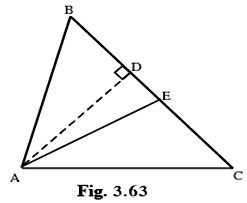Given: AE is bisector of ∠CAB.

To Prove: ∠DAE = 1/2 (∠B - ∠C)

Proof:

We know that ∠BAE = 1/2 ∠A (1)

∠BAD = 90° - ∠B ……………..(2)

On putting equations (1) and (2)

= 1/2 ∠A - (90° - ∠B)

= 1/2 ∠A – 90° + ∠B

= 1/2 ∠A - 1/2 (∠C + ∠A + ∠B) + ∠B

= 1/2 ∠A - 1/2 ∠A - 1/2 ∠B – 1/2 ∠C + ∠B

= 1/2 ∠B – 1/2 ∠C

∴ ∠DAE = 1/2 (∠B - ∠C)

## PDF FILE TO YOUR EMAIL IMMEDIATELY PURCHASE NOTES & PAPER SOLUTION. @ Rs. 50/- each (GST extra)

SUBJECTS

HINDI ENTIRE PAPER SOLUTION

MARATHI PAPER SOLUTION
SSC MATHS I PAPER SOLUTION
SSC MATHS II PAPER SOLUTION
SSC SCIENCE I PAPER SOLUTION
SSC SCIENCE II PAPER SOLUTION
SSC ENGLISH PAPER SOLUTION
SSC & HSC ENGLISH WRITING SKILL
HSC ACCOUNTS NOTES
HSC OCM NOTES
HSC ECONOMICS NOTES
HSC SECRETARIAL PRACTICE NOTES

2019 Board Paper Solution

HSC ENGLISH SET A 2019 21st February, 2019

HSC ENGLISH SET B 2019 21st February, 2019

HSC ENGLISH SET C 2019 21st February, 2019

HSC ENGLISH SET D 2019 21st February, 2019

SECRETARIAL PRACTICE (S.P) 2019 25th February, 2019

HSC XII PHYSICS 2019 25th February, 2019

CHEMISTRY XII HSC SOLUTION 27th, February, 2019

OCM PAPER SOLUTION 2019 27th, February, 2019

HSC MATHS PAPER SOLUTION COMMERCE, 2nd March, 2019

HSC MATHS PAPER SOLUTION SCIENCE 2nd, March, 2019

SSC ENGLISH STD 10 5TH MARCH, 2019.

HSC XII ACCOUNTS 2019 6th March, 2019

HSC XII BIOLOGY 2019 6TH March, 2019

HSC XII ECONOMICS 9Th March 2019

SSC Maths I March 2019 Solution 10th Standard11th, March, 2019

SSC MATHS II MARCH 2019 SOLUTION 10TH STD.13th March, 2019

SSC SCIENCE I MARCH 2019 SOLUTION 10TH STD. 15th March, 2019.

SSC SCIENCE II MARCH 2019 SOLUTION 10TH STD. 18th March, 2019.

SSC SOCIAL SCIENCE I MARCH 2019 SOLUTION20th March, 2019

SSC SOCIAL SCIENCE II MARCH 2019 SOLUTION, 22nd March, 2019

XII CBSE - BOARD - MARCH - 2019 ENGLISH - QP + SOLUTIONS, 2nd March, 2019

HSC Maharashtra Board Papers 2020

(Std 12th English Medium)

HSC ECONOMICS MARCH 2020

HSC OCM MARCH 2020

HSC ACCOUNTS MARCH 2020

HSC S.P. MARCH 2020

HSC ENGLISH MARCH 2020

HSC HINDI MARCH 2020

HSC MARATHI MARCH 2020

HSC MATHS MARCH 2020

SSC Maharashtra Board Papers 2020

(Std 10th English Medium)

English MARCH 2020

HindI MARCH 2020

Hindi (Composite) MARCH 2020

Marathi MARCH 2020

Mathematics (Paper 1) MARCH 2020

Mathematics (Paper 2) MARCH 2020

Sanskrit MARCH 2020

Important-formula

THANKS